﻿ Cartridge Lifting MechanismPublications are Open
Access in this journal
Article Versions
Export Article
• Normal Style
• MLA Style
• APA Style
• Chicago Style
Research Article
Open Access Peer-reviewed

### Cartridge Lifting Mechanism

Darina Hroncová , Jozef Kostka
American Journal of Mechanical Engineering. 2017, 5(6), 257-262. DOI: 10.12691/ajme-5-6-5
Published online: December 15, 2017

### Abstract

The paper gives a basic division of planar mechanisms especially lifting mechanisms. It deals with the analysis of the lifting mechanism of a cartridge. The mechanism is first analyzed analytically using the vector method. The equations of the motion are assembled. Subsequently, the lifting mechanism model is simulated in MSC Adams. The result of the analysis is the graphical representation of calculated kinematic quantities, their dependencies and the evaluation of the results.

### 1. Introduction

A huge amount of material, either new or re-used, has to be loaded into the melting furnaces in the high-pressure die-casting plant during continuous operation. It is quite difficult for a worker to do this. As a consequence replacing this activity introduces a lifting mechanism that makes the process more efficient. The cartridge of the lifting mechanism is filled continuously by a forklift, as the mechanism is on a platform approximately one meter high and works semi-automatically with participation of a worker. When the cartridge is full, the worker lowers the mechanism to the unloading position and if necessary directs the material by the appropriate tools to the melting furnace opening. It is very important that the cartridge position is correct and the material moves in the right direction. The kinematic analysis of the cartridge lifting mechanism is therefore performed.

Firstly we present the division of different mechanisms and an overview of methods of analytic analysis of their kinematics. The most suitable approach is then selected for the subsequent analysis. Computer simulation in MSC Adams is then carried out. The results of both approaches are then compared and evaluated.

### 2. Mechanisms

By a mechanism we mean a mechanical device that is used either to transfer forces or to transform the movement (i.e., to change one type of motion to another).

Mechanisms of various kinds are known 1. Different machines and devices use different kinds of mechanisms but their basic functions are the same 2.

2.1. Planar Mechanisms

Two members which are in contact and can move relative to each other form a kinematic pair 3. The contact of the members can be in a point, a curve or a surface. These are also the elements of the pair. There are so called lower kinematic pairs (the contact of the members is an area) and higher (the contact is in form of a point or a curve).

• Figure 1. Three-member mechanisms
• Figure 2. Four-member mechanisms
• Figure 3. Cam mechanisms
• Figure 4. a) Slot mechanism with a characteristic rapid reverse motion of the member 6, b) kinematic diagram of the OHV mechanism
• Figure 5. Hydraulic Excavator Mechanism

The planar kinematic pairs include rotary, sliding, rolling and general pairs. Different types of mechanisms are showed in (Figure 1), (Figure 2) and (Figure 3) 4.

Composite mechanisms with different applications are in (Figure 4) and (Figure 5) 5.

2.2. Lifting Mechanisms

Some types of planar lifting mechanisms are shown next - the hydraulic lifting mechanism of a truck (Figure 6), the tilting mechanism driven by a hydraulic cylinder (Figure 7) and the mechanism of a load-moving device (Figure 8) 6, 7.

• Figure 6. Hydraulic lifting mechanism of a truck
• Figure 7. Tilting mechanism driven by hydraulic cylinder
• Figure 8. Mechanism of a load-moving device
2.3. Cartridge Lifting Mechanism

The goal is to solve the movement of the cartridge center of gravity and the overall movement of the lifting mechanism as shown in the following (Figure 9) and (Figure 10).

• Figure 9. Cartridge lifting mechanism used in production
• Figure 10. The lifting mechanism in the position when the material is loaded into the melting furnace

### 3. Analytic Analysis

Kinematic analysis of a mechanism describes the motion of each member and its characteristic points resulting of the movement of the driven members 8.

It is necessary to determine the dependency of position, speed and acceleration of the examined members and points on the position of the driven members or on time, and other dependencies derived from them 4. The analytic analysis is carried out on a planar lifting mechanism (Figure 10).

3.1. Vector Method

The vector method is a general method that is useful for kinematic investigation of planar mechanisms. This method characterizes the kinematic scheme of the mechanism by means of vector polygons and thus provides general guidance on how to proceed to equations describing the position, velocity and acceleration of the mechanism 4.

If the mechanism is simple it is described by one vector polygon. In case of complex ones, several polygons are required 5. The individual sides of the polygon are vectors oriented one after the other so that the closure condition for the polygon stands.

• Figure 11. Schema of the lifting mechanism

After defining the vectors in the graphical model of the mechanism (Figure 11) and the vector polygons, vector equations are then created 9. These are then rewritten into two scalar equations. Then a substitution is introduced which simplifies the solution and makes it more transparent. First we determine the angle of rotation of the cartridge φ31, mark the length AB = l*. The lifting mechanism has 2 degrees of freedom. Analysis is based on the OABS shape closure condition.

For the polygon (Figure 11) the vector equation applies: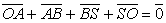(1)

Rewriting the vector equation in the x and y axes:(2)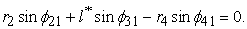(3)

We need to specify the angle φ31, so we transform the equations as follows: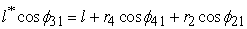(4)(5)

By modification: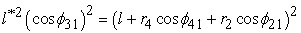(6)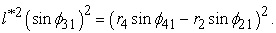(7)(8)

From equations (4) and (5) we determine the angle φ31.

For determination of the position of the center of gravity the vector equation applies: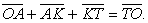(9)

Rewriting the vector equation in the x and y axes:(10)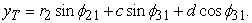(11)

There are the equations (10) and (11) of center of gravity positions xT and yT. The location of the cartridge center in time (t=1 second) is in (Table 5). After derivation we obtain the equations of x and y components of the velocities and the acceleration of the center of gravity in (Table 5) 5.

### 4. Computer Simulation

MSC Adams gives engineers the opportunity of verifying the design on a computer model reducing design cost and increasing work efficiency 12. This simulation can evaluate and control complex interactions between bodies including their movement 10. The modeling of the mechanism and the evaluation of the results are shown in successive steps in the following chapters.

4.1. Modeling of the Mechanism

We simulated the mechanism according to its exact dimensions. First we created the cranks 2 and 4 (Figure 11a), then the roller and finally the cartridge which consists of three smaller parts (Figure 12b).

• Figure 12. Modeling steps

We used rotational and sliding links to connect the respective parts (Figure 13a,b). Finally we defined the actuators (Figure 13c) and verified the model 11.

• Figure 13. Modeling steps

The mechanism performed the predicted motion. As an example we are giving an overview of some modeling steps in picture (Figure 14a, b).

• Figure 14. Model of the mechanism

### 5. Simulation Results

Numerical results of the simulation can be acquired from the model by using so called “meters”. They provide the kinematic and dynamic quantities in respective members of the model. These quantities for selected model parts are shown in the following picture (Figure 15).

• Figure 15. Rotations and distances a) angle of crank 2, b) angle of crank 4, c) displacement l*

Our goal is to investigate the movement of the center of gravity of the cartridge. After inserting the appropriate meter we obtain its position, velocity and acceleration. Respective quantities are shown in the picture (Figure 16).

• Figure 16. The result parameters a) position xT and yT, b) velocity vxT and vyT, c) acceleration axT and ayT
• Figure 17. The result parameters a) velocity vT, b) acceleration aT
• Figure 18. The result parameters a) velocity vT=f(sT), b) velocity aT=f(sT), c) acceleration aT=f(vT)
• Figure 19. The result parameters a) position sT=f(φ21), b) velocity vT=f(φ41), c) acceleration aT=f(φ21)
5.1. Result Evaluation

Simulation in MSC Adams and results processing gave us the specific values which represent the movement of the center of the cartridge (Table 5).

The cranks 2 and 4 perform a rotary motion around the points we have defined and they rotate at the same angular speed. The roller (member 5) performs a sliding motion in the hollow cylinder that is part of the cartridge. The movement of the cartridge is composed of two rotating and one sliding movement. We've found that the cartridge's center of gravity performs an accelerated movement along a curve. Both the velocity (Tab.2) and acceleration (Tab.3) components in the y-axis direction are larger than the velocity and acceleration components in the x-axis direction, so it is obvious that the cartridge is more lifted than shifted. The fact that the cartridge is lifted follows from comparison of the coordinates xT and yT of the initial state and the state in the investigated position.

### 6. Conclusion

We investigated the movement of the center of gravity and the overall movement of the lifting mechanism. The solution was accomplished by two methods, analytically and by computer simulation. The vector method was used as an analytic method. The computer simulation was performed by MSC Adams. The results of the simulation are presented in form of graphs and tables with quantities of the investigated model parts.

The results of both solutions match. The simulation in MSC Adams is however faster and so more effective.

### Acknowledgements

This work was supported by grant projects VEGA 1/0872/16 Research of synthetic and biological inspired locomotion of mechatronic systems in rugged terrain, grant projects VEGA 1/0751/16 and grant projects VEGA 1/0731/16 Development of advanced numerical and experimental methods for the analysis of mechanical systems.

### References

  Jančina, J., Pekárek, F.: Mechanika II., Kinematika, Alfa, , 1987. In article  Brát, V.: Příručka kinematiky s příklady, SNTL/Alfa, Praha, 1973. In article  Brát, V., Jáč, V., Rosemberg, J.: Kinematika, SNTL/Alfa, Praha, 1964. In article PubMed  Juliš, K., Brepta, R.: Mechanika I, Statika a kinematika, SNTL, Praha, 1986. In article  Caban, S., Chlebová Z.: Kinematika. Košice, 2006. In article  Columbyová, K., Chlebová Z., Blanár J.: Mechanika II. VST, Košice, Slovakia, 1989. In article  Bradský, Z., Jáč, V.: Mechanika II., Kinematika. Edičné stredisko Liberec, 1979. In article  Myszka David H.: Machines and mechanisms, applied kinematic analysis, 2012. In article  Kostka, J.: Kinematická analýza a počítačová simulácia zdvíhacieho mechanizmu zásobníka, Kinematic analysis and computer simulation of container lifting mechanism. Bakalárska práca, SjF TU, Košice, Slovakia, 2017. In article  Delyová, I., Frankovský, P., Hroncová, D., Kinematic analysis of movement of a point of a simple mechanism,” 4th International Conference Modelling of mechanical and mechatronics systems, KAMaM, Technical university Košice, Herľany, Slovakia, 2011. In article  Virgala, I., Frankovský, P., Kenderová, M.: Friction Effect Analysis of a DC Motor. In: American Journal of Mechanical Engineering, Vol. 1, No. 1, p. 1-5, 2013. In article View Article  http://www.mscsoftware.com/product/adams. In article View ArticleThis work is licensed under a Creative Commons Attribution 4.0 International License. To view a copy of this license, visit http://creativecommons.org/licenses/by/4.0/